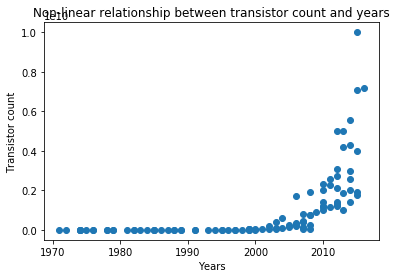# Implementing Moore’s Law Using PythonHi All! Today, we will learn how to implement Moore’s Law Using Linear Regression in Python. Before going through this tutorial, it is highly recommended to go through the mathematics behind this tutorial.

Moore’s law states that in an Integrated Circuit, the transistor count doubles every year or transistor count, Y = a*2^X where a is any arbitrary constant and X is the time in years.Below is the implementation of this concept.

In :
```import re                                 #importing package for regular expressions
import numpy as np                        #import numpy to make matrix and vector computations easier
import matplotlib.pyplot as plt           #import matplotlib.pyplot to visualize the vectors on graphs

X_input = []                                    #making empty X_input list for the input vector
Y_output = []                                    #making empty Y_output list for the output vector

non_dec = re.compile(r'[^\d]+')       #using regular expressions for splitting the data and then substituting it with other values
for l in open("moore_law.csv"):
reg = l.split('\t')
x = int(non_dec.sub('', reg.split('[')))
y = int(non_dec.sub('', reg.split('[')))

X_input.append(x)
Y_output.append(y)

X_input = np.array(X_input)         #converting X_input into numpy array
Y_output = np.array(Y_output)       #converting Y_output into numpy array

plt.scatter(X_input, Y_output)      #making scatter plot of X_input and Y_output to see the relationship between them
plt.title('Non-linear relationship between transistor count and years')
plt.xlabel('Years')
plt.ylabel('Transistor count')
plt.show()

Y_output = np.log(Y_output)   #Since Y_output = a*2^X_input, taking the log of Y_output and then drawing the scatter plot of log(Y_output) and X_input.
plt.scatter(X_input, Y_output)
plt.title('After taking log, graph turns out to be linear')
plt.xlabel('Years')
plt.ylabel('log of Transistor count')
plt.show()

denominator = X_input.dot(X_input) - X_input.mean()*X_input.sum()       #Calculating m and c and then finding predicted Y or Yhat
m = (X_input.dot(Y_output)-Y_output.mean()*X_input.sum())/denominator
c = (Y_output.mean()*X_input.dot(X_input) - X_input.mean()*X_input.dot(Y_output))/denominator

Yhat = m*X_input + c                            #Calculating Yhat

plt.scatter(X_input, Y_output)
plt.plot(X_input, Yhat, color='red')
plt.show()

diff1 = Y_output - Yhat                          #Calculating Rsquare
diff2 = Y_output - Y_output.mean()
rsquare = 1 - (diff1.dot(diff1)/diff2.dot(diff2))
print("m:", m, "c:", c)
print("The R squared is:", rsquare)

print("time to double is ", np.log(2)/m, "years")
``````m: 0.351043573365 c: -685.000284382
The R squared is: 0.952944285229
time to double is 1.97453317238 years
```